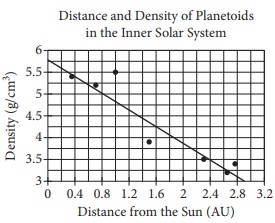mycollegehive
The scatterplot above shows the densities of 7 planetoids,...
SAT-math-test SAT math0The scatterplot above shows the densities of 7 planetoids, in grams per cubic centimeter, with respect to their average distances from the Sun in astronomical units (AU). The line of best fit is also shown.

According to the scatterplot, which of the following statements is true about the relationship between a planetoid’s average distance from the Sun and its density?

A) Planetoids that are more distant from the Sun tend to have lesser densities.

B) Planetoids that are more distant from the Sun tend to have greater densities.

C) The density of a planetoid that is twice as far from the Sun as another planetoid is half the density of that other planetoid.

D) The distance from a planetoid to the Sun is unrelated to its density

841 viewsShareFollowAsked by judziyrep:1.9K
University of Benin Nigeria
30 May 2020

Comments

### 1 answer(s)

Advertisement (continue below)Peter answeredrep:1.7K
University of Lagos Nigeria
25 October 20200From the graph, it is seen that the density decreases as the distance increases

Ans AShare
Comments

Advertisement (continue below)

### Groups

Advertisement (continue below)

Your Answer

How to insert math formulas/equations### Related Tags

SAT-math-test

13 followers

476 questionsSAT

7 followers

487 questionsmath

20 followers

1261 questions